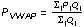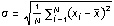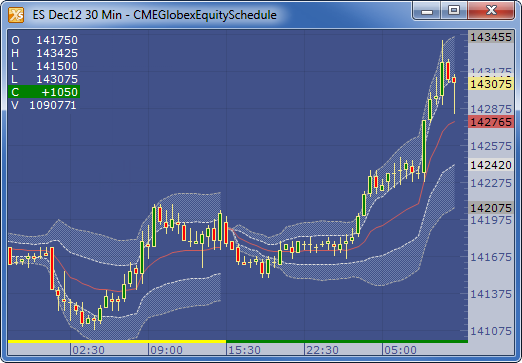← Back to X_TRADER® Help Library

### X_STUDY® Documentation

#### Volume Weighted Average Price (VWAP)

##### Description

The Volume Weighted Average Price is similar to a moving average, except volume is included to weight the average price over a one day period. VWAP resets daily and can be calculated based on exchange session, primary session and custom defined sessions. You can also apply standard deviation bands above and below the VWAP.

In the example below the VWAP line is red with two standard deviations bands above and below. Shading between the bands has been applied to highlight this region.

Note: You cannot apply the VWAP technical indicator to Price Distribution and Tick charts. VWAP applied to a spread chart is based on price updates to the spread chart. Each price update on either leg of a spread chart is assigned a volume value of one.

##### Formulawhere:

• PVWAP = Volume Weighted Average Price
• Pj = price of trade j
• Qj = quantity of trade j
• j = each individual trade that takes place over the defined period of time.

To calculate the standard deviation value to place the upper and lower channel lines use the following formula:Middle Band = Pvwap

Upper Band = Middle Band + (x) Standard Deviation

Lower Band = Middle Band - (x) Standard Deviation

Note: For the VWAP standard deviation calculation, X represents the VWAP value calculated at each bar and x is the average of the VWAP since the session start. The standard deviation will be zero on the first bar of each session since ( xi - x ) will be zero and N is one.